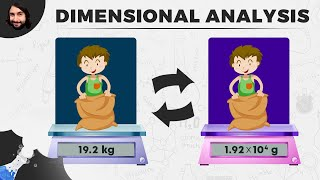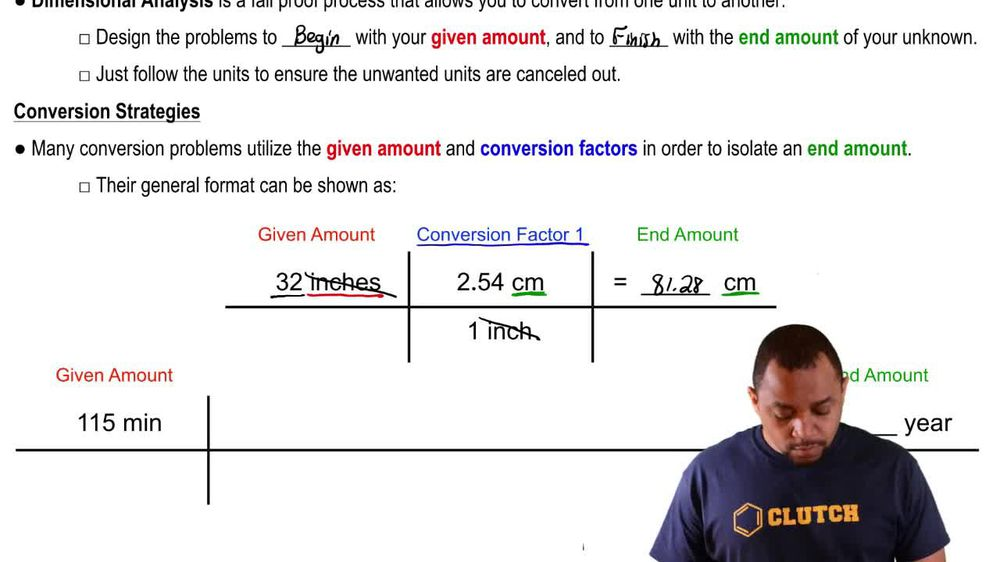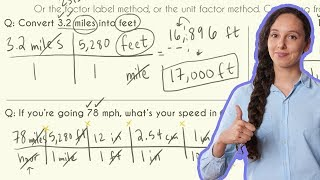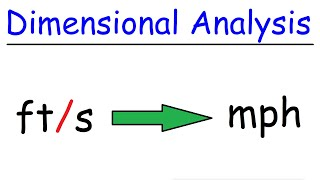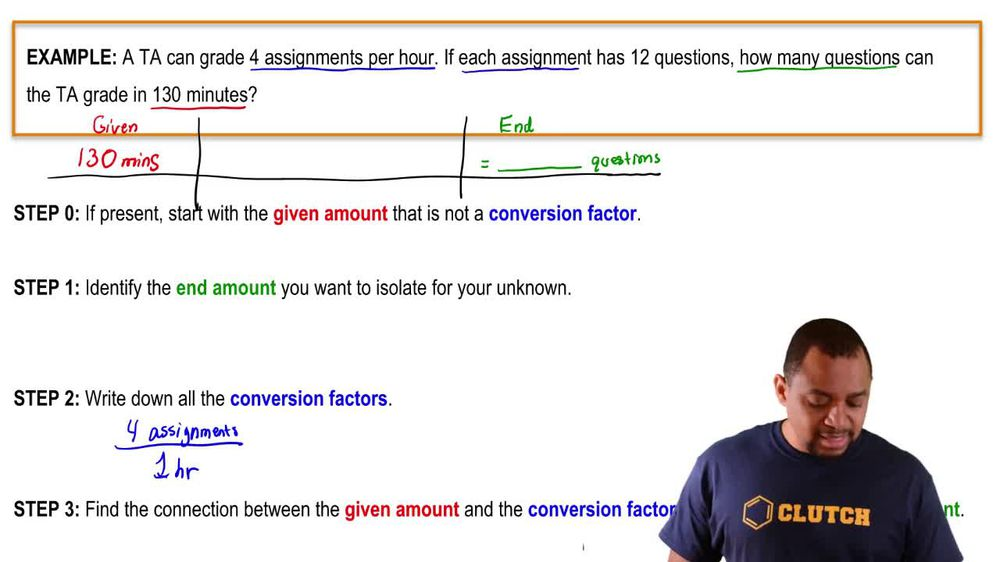Start typing, then use the up and down arrows to select an option from the list.
1. 1. Intro to General Chemistry2. Dimensional Analysis
Problem

# Concentrations of substances dissolved in solution are often expressed as mass per unit volume. For example, normal human blood has a cholesterol concentration of about 200 mg/100 mL. Express this concentration in the following units. (e) How much total blood cholesterol in grams does a per-son have if the normal blood volume in the body is 5 L?

Relevant Solution1m
Play a video:
Hello everyone today we are being given the falling problem, diabetes is usually tested by measure measuring fasting blood glucose levels. A person was found to have a fasting blood glucose level of a 120 mg per deciliter, Calculate the fasting blood glucose in grams of this person if the total blood volume of this person is 5.7 L. So the first thing you wanna do is you want to start with our given which is our 5.7 L. When you want to somehow convert this, Using the conversion factor of that Milligrams over one deciliter. We want to also multiply by the conversion factor that 10 to the third grams or negative third grams is equal to one mg. And then to get rid of that desolate er we simply use the conversion factor that one desolate is equal to 10 to the negative one leader. And so this gets rid of escalators and leaders. And so we're just left with g. This gives us 6.8 g as our final answer. And with that we've answered the question. I hope this helped. And until next time